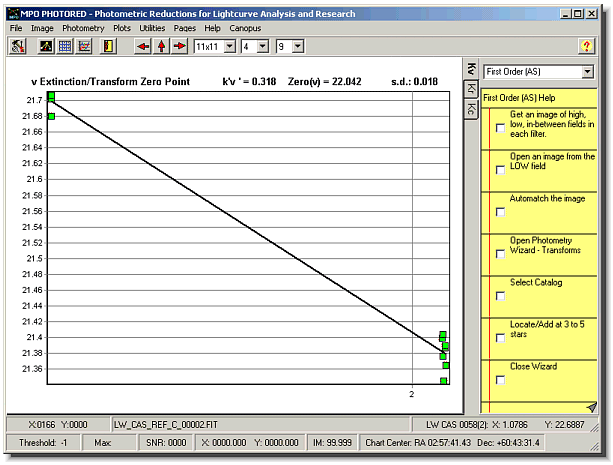# The Minor Planet Observer andPalmer Divide Observatory

(opens new window)

MPO Support

CALL web site

Society for Astronomical Sciences

99999999999999999999

Home
Up

# PhotoRed Reductions Routines - First Order (Modified Hardie)Hardie explained this method in his section of Astronomical Techniques edited by W.A. Hiltner. It is particularly useful because it requires a minimum of time and effort to obtain the first order extinction values, leaving more time for observing the selected target. It also avoids problems due to changing conditions.

The Hardie method relies on the difference between the actual measured magnitudes of two widely separated stars versus the difference in their true magnitudes. For example, if Star 1 and Star 2 differ by 1.0 magnitude in the catalog and differ by 1.2 in air mass, one would expect the difference in the measured magnitudes to be 1.0 (on the assumption that there is no extinction). However, the measured difference will not be 1.0 but a little more or less because of the air mass difference.

Stated mathematically

`k’ = ((M1 - M2) - (m1 - m2)) / (X1 - X2)`
```where   M1   catalog magnitude of Star 1
M2   catalog magnitude of Star 2
m1   measured magnitude of Star 1
m2   measured magnitude of Star 2
X1   air mass for Star 1
X2   air mass for Star 2```

The PhotoRed routine modifies this approach somewhat and also accounts for an assumption made in Hardie’s original paper. Specifically, that the instrumental magnitudes were on the standard color system. Naturally, raw uncorrected values measured from the images are not. In order to make that true, one must first find the transforms for the colors being used. These are applied to each raw instrumental magnitude – without any correction for extinction, of course, before being put into a least squares solution.

Lacking any atmospheric correction, the derived magnitude values in both fields would cluster around a constant value. Why? Say that a 10th magnitude star has a corrected instrumental magnitude of –10. In theory, a 9th magnitude star of exactly the same color (or adjusted to the same color by the transform), will have a transformed instrumental magnitude of –11 (remember that instrumental magnitudes are like the star magnitudes in that the brighter the star, the more negative the magnitude). The difference between these two pairs is 20. This is demonstrated in the plot above where the data points at each air mass are within a range of <0.1m. The introduction of extinction changes the constant value for each field, with the higher field’s constant difference being larger because the instrumental magnitudes are a bit brighter (more negative).

Using the averages of the derived values in each filed, PhotoRed finds the slope using a least squares solution and the formula

`k’ = (Avg1 – Avg2) / (X1 – X2)`

where Avg1 and X1 represent the field with the higher air mass (lower altitude)

## Requirements for the Method

1. Conditions be as identical as possible when you take the images to be measured, e.g., don’t shoot one in a thin haze.
2. All stars being measured must have catalog values for the two filters you use to take the images. Don’t make the mistake of selecting the V-I color index only to find that one of the fields has no I catalog magnitudes.
3. The air mass difference should be as large as possible, at least 0.5 and preferably 0.7 to 1.0. This would mean shooting a reference field near the zenith and one about 30° above the horizon.

 This site is best viewed at 1024x768 and higher This page was last updated on 01/19/11 05:14 -0700. All contents copyright (c) 2005-2011, Brian D. Warner Trademarks are the property of their respective owners